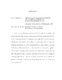## Transfer of Representations and Orbital Integrals of Inner Forms of GL_n2016
##### Authors
Cohen, Jonathan
Let $F$ be a nonarchimedean local field and $D$ an $F$-central division algebra. We characterize the Local Langlands Correspondence (LLC) for inner forms of $GL_n$ over $F$ via the Jacquet-Langlands Correspondence and compatibility with the Langlands Classification. We show that LLC satisfies a natural compatibility with parabolic induction and characterize the LLC for inner forms as a unique family of bijections $\Pi(GL_r(D)) \rightarrow \Phi(GL_r(D))$ for each $r$, (for a fixed $D$) satisfying certain properties. We construct a surjective map of Bernstein centers $\mathfrak{Z}(GL_n(F))\to \mathfrak{Z}(GL_r(D))$ and show this produces pairs of matching distributions in the sense of \cite{SBC}. Finally, we construct explicit Iwahori-biinvariant matching functions for unit elements in the parahoric Hecke algebras of $GL_r(D)$, and thereby produce many explicit pairs of matching functions.